# Examples NOT using cosine rule

If the following questions came up in the exam we recommend using LOGIC to work out how to solve this problem using standard trigonometry (SOH CAH TOA) and Pythagoras.

Example 1

What is the length Q to R in the following diagram?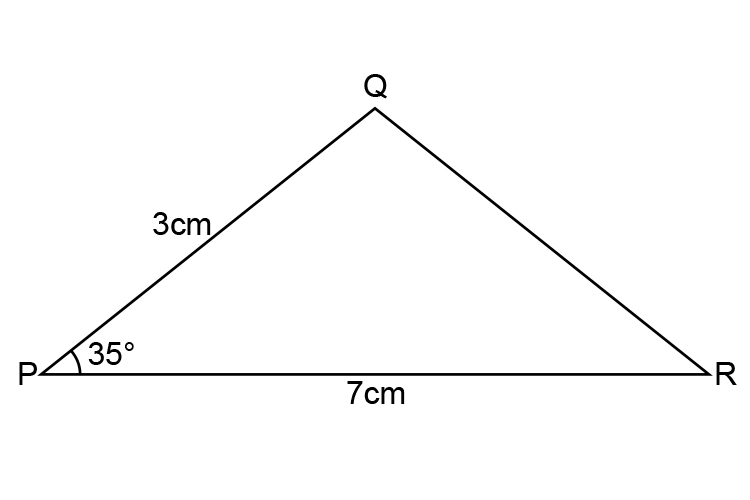To solve this redraw the diagram as: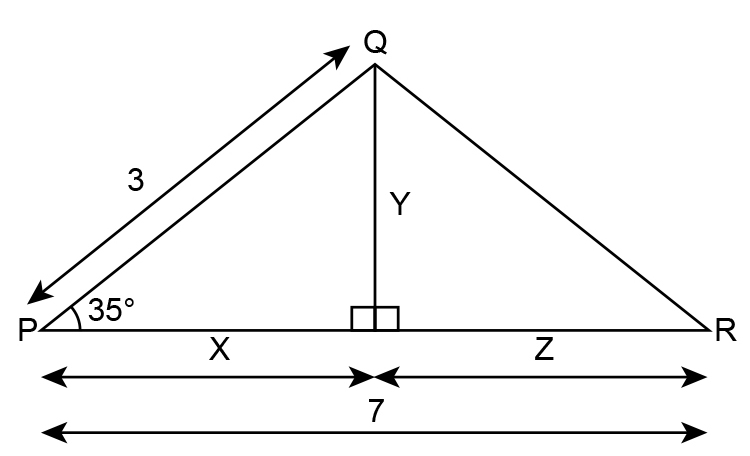Use trigonometry (SOH CAH TOA) to find the value of x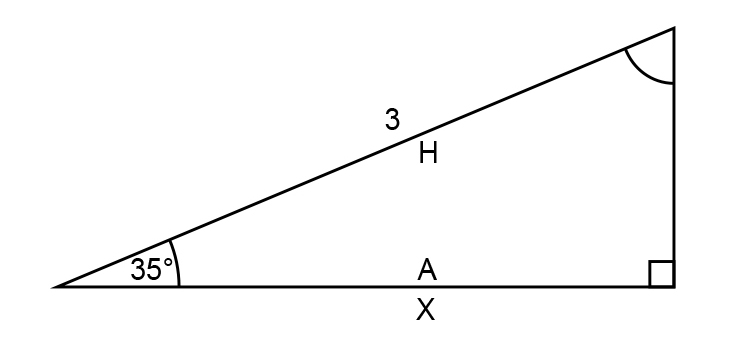costheta=a/H

cos35^@=x/3

x=cos35^@times3

x=0.8191times3=2.457

x=2.457

Now use Pythagoras’s theorem to find Y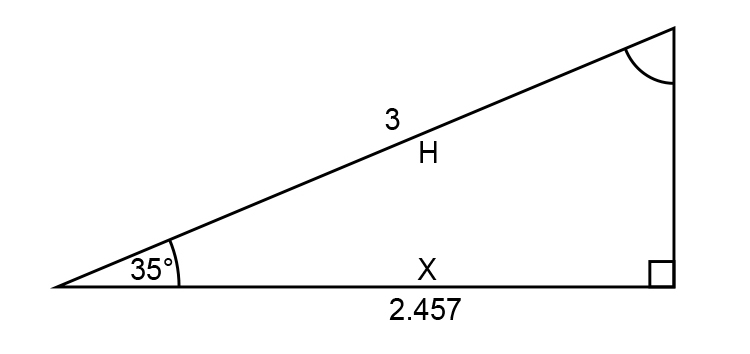H^2=x^2+Y^2

3^2=2.457^2+Y^2

Y^2=3^2-2.457^2

Y^2=9-6.0368

Y^2=2.9631

Y=sqrt 2.9631

Y=1.721

Now find z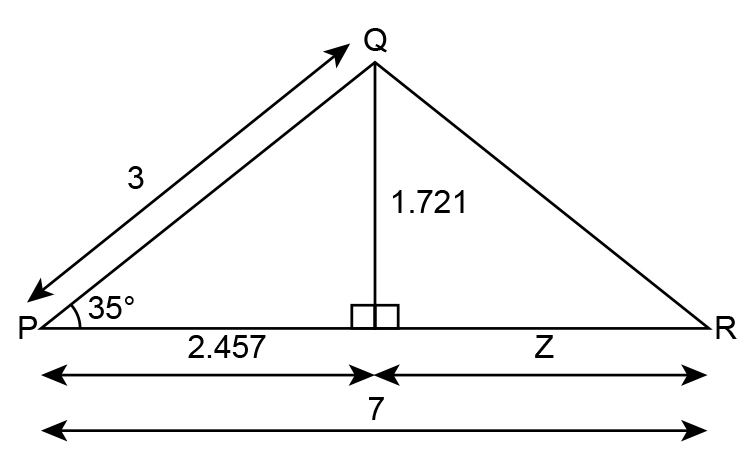z is simply 7-2.457=4.543

z=4.543

Then use Pythagoras’s theorem to find QR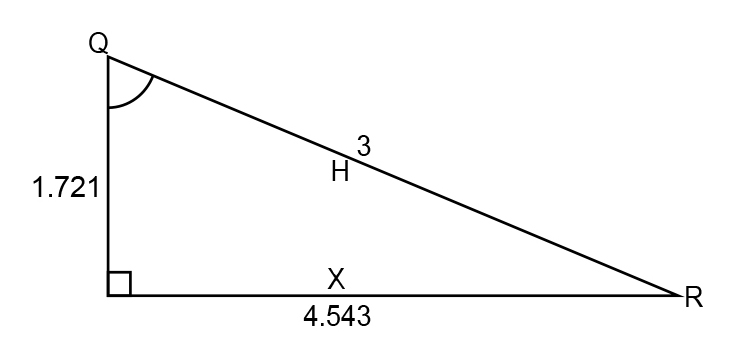H^2=x^2+y^2

QR^2=4.543^2+1.721^2

QR^2=20.638+2.961

QR^2=23.59

QR=sqrt 23.59

QR=4.58cm

NOTE:

This may look long winded but it is incredibly logical based on the knowledge you should already have on Pythagoras and trigonometry.

Example 2

In the diagram below find theta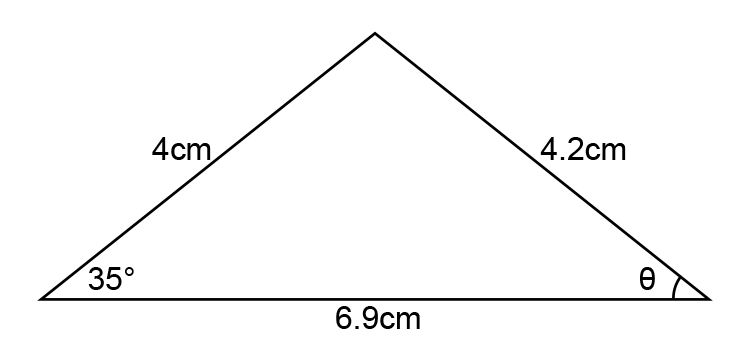Redraw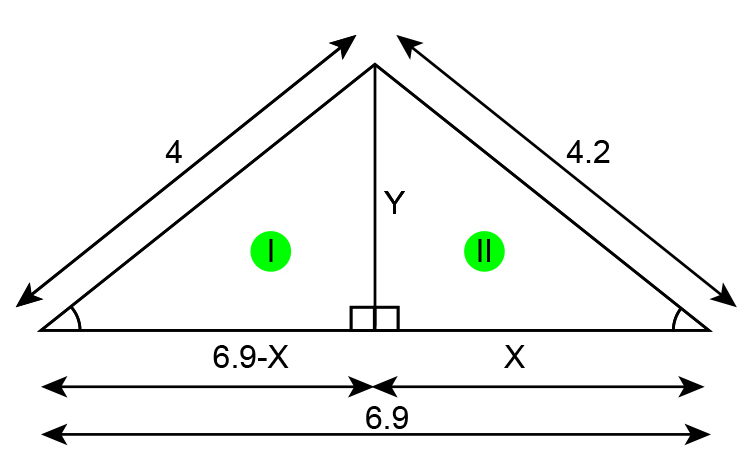First find x for triangle I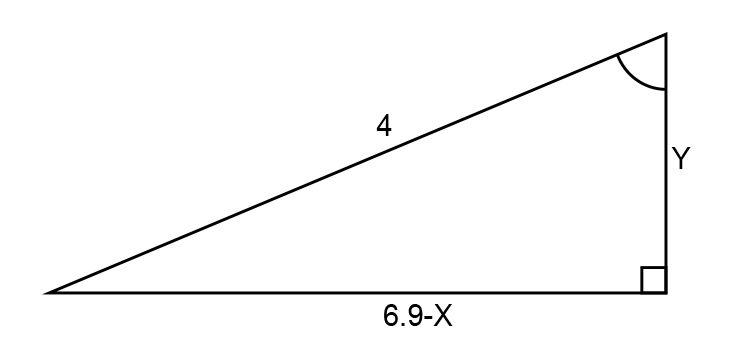Using Pythagoras’s theorem:

4^2=(6.9-x)^2+Y^2

4^2=6.9^2-6.9x-6.9x+x^2+Y^2

16=47.61-13.8x+x^2+Y^2

16-47.61+13.8x-x^2=Y^2

-31.61+13.8x-x^2=Y^2…………… Equation 1

For triangle II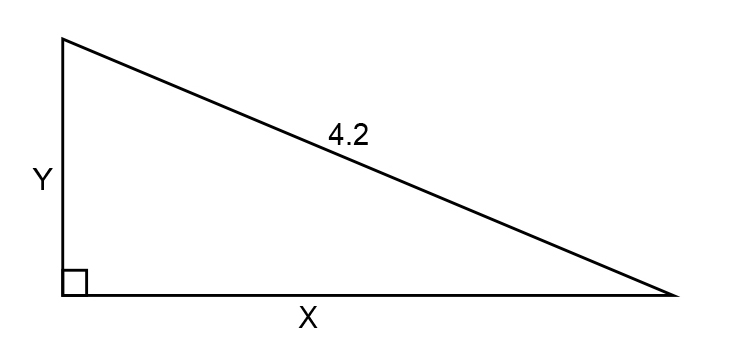Y^2+x^2=4.2^2

Therefore Y^2=4.2^2-x^2 ……………. Equation 2

Substitute equation 2 into equation 1

-31.61+13.8x-x^2=4.2^2-x^2

-31.61+13.8x=4.2^2-x^2+x^2

-31.61+13.8x=4.2^2

13.8x=4.2^2+31.61

x=(4.2^2+31.61)/13.8

x=3.56cm long

Now use cosine on triangle II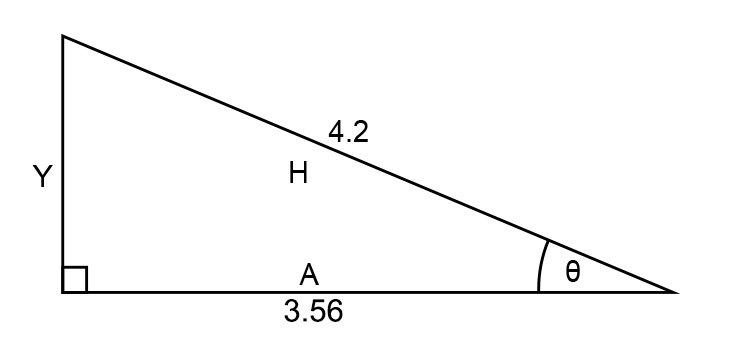Find theta

costheta=3.56/4.2

costheta=0.849

theta=31.8^@

Again this may look long winded but the example can be completed without the need of using the cosine rule, it just takes practice.